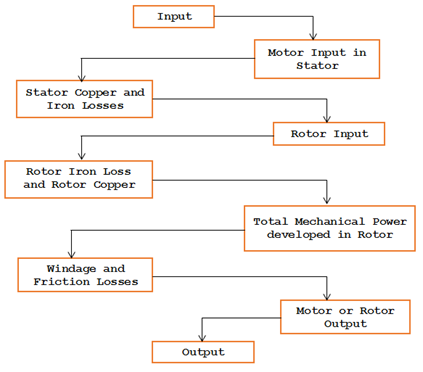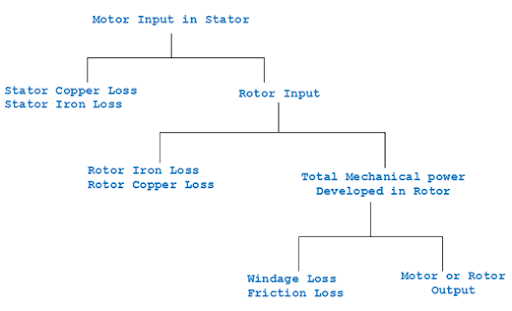# Induction Motor Output, Losses and Efficiency

The various losses in the stator of three phase induction motor are stator copper losses and stator iron losses. The stator iron losses consist of eddy current loss and hysteresis loss.

In rotor the losses are rotor iron loss and rotor copper loss. The other losses on the rotor side are friction losses at bearing and slip rings etc and the windage losses.

The stator iron losses depend upon the two factors namely flux density and the supply frequency. The rotor iron loss depends on the frequency of the rotor current. The frequency of the rotor current depends on the slip or speed of the rotor (f=sf). The iron losses of the rotor are negligible, since the frequency of the rotor current is very small.

#### Power stages and various losses in an Induction Motor:#### Power flow chart of an Induction Motor:1. Rotor copper loss is determined by using the formula 3I22R2.
2. Stator copper loss is calculate by using the formula 3I21R1
3. Input to the motor on load is equal to √3VLIL cosΦ watts.

### Three Phase Induction Motor Rotor output:

In an induction motor is supplied to the stator winding. The power to the rotor circuit is transferred entirely by induction. The output available at the rotor shaft is mechanical energy.

During the power conversion, certain losses take place at various stages. The rotor output of an induction motor is given as follows:
Stator output = Stator input – stator losses
Stator output = Rotor Input
Rotor Output = Rotor Input – Rotor copper losses
Rotor output = Mechanical Energy
Mechanical Energy ----> Gross Torque Tg

Gross Torque Tg = (useful torque or shaft torque Tsh) + (Winding and friction losses in the motor)
Rotor output = 2πNrTg

Where, Nr = actual speed of the motor in r.p.m
Tg = Gross torque in NM
From this Tg = Rotor output in watts / 2πN--------> 1

For an ideal case, assuming no copper losses in the rotor circuit.
Rotor Output = rotor Input
For this condition, the motor should run at synchronous speed.
Then the torque,
Tg = Rotor Input / 2πN--------> 2
Using the eqn 1 & 2
Rotor output = 2πNrT--------> 3
Rotor input 2πNsT--------> 4

But in actual case
(Rotor input – Rotor output) = Rotor copper losses.
using the eqn 3 & 4
(2πNsTg - 2πNrTg) = Rotor copper losses.
That is Rotor copper losses = Tg. 2π(Ns – Nr)   --------> 5
Using the eqn 4&5
Rotor copper losses/Rotor input = Tg2π(Ns-Nr) / 2πNrsTg
= (Ns-Nr) / Ns
= Slip S  --------> 6
Thus , from the equation 6, Rotor copper losses equal to
s x Rotor input   --------> 7
But Rotor Gross Output = Rotor input – Rotor copper losses
Using equation 7
Rotor gross output = (Rotor input – s) x Rotor input
= (1-s) Rotor input
Or

Rotor gross output/Rotor input = (1-s)  --------> 8
Using s, (Ns – Nr) /Ns
Rotor gross output/Rotor input = {1- (Ns – Nr) /Ns}
= (Ns-Ns+Nr) / Ns
=Nr/Ns
That is,
Rotor gross output/Rotor input = Actual speed of the motor / Synchronous speed
Hence this can be expressed as
Rotor efficiency = Nr/Ns

Using the expression (6 and 8), (6+8) gives
Rotor copper losses/Rotor Gross input = s/(1-s)

### Efficiency of an Induction Motor

The induction motor receives power from the supply mains through the stator windings. The input power is electrical power. The power from the stator winding is transferred to rotor, via air gap by induction principle. The output power is a mechanical power. The mechanical power is obtained at the shaft of the motor to perform mechanical work.

During this power transfer, some losses such as copper losses in stator winding, copper losses in rotor winding, core losses in stator winding, frictional losses and windage losses take place.

Then the efficiency is determined by knowing the input power and the output power.

Efficiency of any machine is the ratio of output power and input power. The efficiency of an induction motor is obtained as

Efficiency η = Output power / input power
= Output power / (Output power + losses)0 CommentsComments